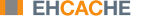Product Documentation : Ehcache Configuration Guide : Sizing Storage Tiers : Eviction When Using CacheManager-Level Storage
Eviction When Using CacheManager-Level Storage
When a CacheManager-level storage pool is exhausted, a cache is selected on which to perform eviction to recover pool space. The eviction from the selected cache is performed using the cache's configured eviction algorithm (LRU, LFU, etc...). The cache from which eviction is performed is selected using the “minimal eviction cost” algorithm described below:
eviction-cost = mean-entry-size * drop-in-hit-rate
Eviction cost is defined as the increase in bytes requested from the underlying SOR (System of Record, e.g., database) per unit time used by evicting the requested number of bytes from the cache.
If we model the hit distribution as a simple power-law then:
P(hit n'th element) ~ 1/n^{alpha}
In the continuous limit, this means the total hit rate is proportional to the integral of this distribution function over the elements in the cache. The change in hit rate due to an eviction is then the integral of this distribution function between the initial size and the final size. Assuming that the eviction size is small compared to the overall cache size, we can model this as:
drop ~ access * 1/x^{alpha} * Delta(x)
where “access” is the overall access rate (hits + misses), and x is a unit-less measure of the “fill level” of the cache. Approximating the fill level as the ratio of hit rate to access rate, and substituting in to the eviction-cost expression, we get:
eviction-cost = mean-entry-size * access * 1/(hits/access)^{alpha}
* (eviction / (byteSize / (hits/access)))
Simplifying:
eviction-cost = (byteSize / countSize) * access * 1/(h/A)^{alpha}
* (eviction * hits)/(access * byteSize)
eviction-cost = (eviction * hits) / (countSize * (hits/access)^{alpha})
Removing the common factor of “eviction”, which is the same in all caches, lead us to evicting from the cache with the minimum value of:
eviction-cost = (hits / countSize) / (hits/access)^{alpha}
When a cache has a zero hit-rate (it is in a pure loading phase), we deviate from this algorithm and allow the cache to occupy 1/nth of the pool space, where “n” is the number of caches using the pool. Once the cache starts to be accessed, we re-adjust to match the actual usage pattern of that cache.|   Feedback